Notes On Introduction to Solid Shapes - CBSE Class 7 Maths
All two-dimensional figures have only length and breadth. e.g. A paper has only length and breadth, and hence, it is classified as a plane or two-dimensional figure. Three-dimensional solid shapes have length, breadth and height. e.g. a box is in three-dimensional shape.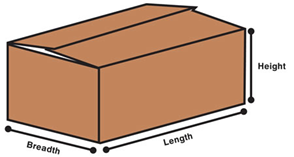Faces The flat surface of a three dimensional shape is called a Face. Edges Two faces of a solid shape meet to form an Edge. Vertices The points where three edges meet are called vertices.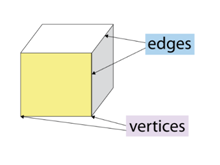The table shows the number of the faces, edges and vertices of some shapes.           Solid shape   Face (F)   Edge (E)   Vertices (V)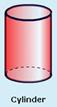3         2           0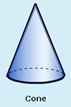2         1           1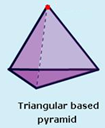4         6           4 Nets of three-dimensional shapes The net of a three-dimensional solid is a two-dimensional skeleton outline, which, when folded, results in the three-dimensional shape.Drawing solid shapes on a flat surface Solid shapes can be drawn on a flat surface, which is known as the two-dimensional representation of a three-dimensional solid. Sketches of solids are of two types: oblique and isometric. Oblique sketches are drawn on squared paper. The oblique sketch gives the visual representation of a solid shaped object but does not the represent the actual dimensions. Isometric sketches are drawn on dotted or isometric sheets and have the exact measurements of solids. The two-dimensional surface on which we draw an image is usually flat. So, when we try drawing a solid shape on a paper or a board, the image appears a little distorted. However, this is just an optical illusion. When look at the picture of the cube. Not all the lines forming the cube are of equal length. Also, we are unable to see all the faces of the cube. In spite of this illusion, we can make out that the image is of a cube. Such skeletons of solids are called oblique sketches. They can be drawn using a squared paper. The first step is to draw the front face of the cube. Then the opposite face of the cube are drawn. This face should also be of the same size as that of the first square. The number of squares is used as a reference for maintaining the size. Now, the corresponding corners of the squares are joined. And finally, the edges that cannot be seen are drawn, with dotted lines. We can see that the front face and the opposite face of the cube are of the same size. Also, the edges appear equal, though we do not draw them of the same length.All of us must have solved puzzles on isometric sheets at some point of time. An isometric sheet divides a screen into small equilateral triangles made of dots. Using this sheet, we can draw sketches with measurements that agree with that of a given solid. Let’s try to draw the sketch of a cuboid of length three units, breadth two units and height two units. First,  a rectangle of length three units and breadth two units is drawn. Then four parallel line segments, each of length two units are drawn starting from the four corners of the rectangle. Finally, the matching corners with appropriate line segments are connected. The isometric sketch of the cuboid is formed. Observe that the measurements are of the exact size, which is not the case with oblique sketches.#### Summary

All two-dimensional figures have only length and breadth. e.g. A paper has only length and breadth, and hence, it is classified as a plane or two-dimensional figure. Three-dimensional solid shapes have length, breadth and height. e.g. a box is in three-dimensional shape.Faces The flat surface of a three dimensional shape is called a Face. Edges Two faces of a solid shape meet to form an Edge. Vertices The points where three edges meet are called vertices.The table shows the number of the faces, edges and vertices of some shapes.           Solid shape   Face (F)   Edge (E)   Vertices (V)3         2           02         1           14         6           4 Nets of three-dimensional shapes The net of a three-dimensional solid is a two-dimensional skeleton outline, which, when folded, results in the three-dimensional shape.Drawing solid shapes on a flat surface Solid shapes can be drawn on a flat surface, which is known as the two-dimensional representation of a three-dimensional solid. Sketches of solids are of two types: oblique and isometric. Oblique sketches are drawn on squared paper. The oblique sketch gives the visual representation of a solid shaped object but does not the represent the actual dimensions. Isometric sketches are drawn on dotted or isometric sheets and have the exact measurements of solids. The two-dimensional surface on which we draw an image is usually flat. So, when we try drawing a solid shape on a paper or a board, the image appears a little distorted. However, this is just an optical illusion. When look at the picture of the cube. Not all the lines forming the cube are of equal length. Also, we are unable to see all the faces of the cube. In spite of this illusion, we can make out that the image is of a cube. Such skeletons of solids are called oblique sketches. They can be drawn using a squared paper. The first step is to draw the front face of the cube. Then the opposite face of the cube are drawn. This face should also be of the same size as that of the first square. The number of squares is used as a reference for maintaining the size. Now, the corresponding corners of the squares are joined. And finally, the edges that cannot be seen are drawn, with dotted lines. We can see that the front face and the opposite face of the cube are of the same size. Also, the edges appear equal, though we do not draw them of the same length.All of us must have solved puzzles on isometric sheets at some point of time. An isometric sheet divides a screen into small equilateral triangles made of dots. Using this sheet, we can draw sketches with measurements that agree with that of a given solid. Let’s try to draw the sketch of a cuboid of length three units, breadth two units and height two units. First,  a rectangle of length three units and breadth two units is drawn. Then four parallel line segments, each of length two units are drawn starting from the four corners of the rectangle. Finally, the matching corners with appropriate line segments are connected. The isometric sketch of the cuboid is formed. Observe that the measurements are of the exact size, which is not the case with oblique sketches.Previous